Processing ......FreeComputerBooks.com Links to Free Computer, Mathematics, Technical Books all over the World

Introduction to Applied Linear Algebra: Vectors, Matrices, and Least Squares
Top Free JavaScript Books 🌠 - 100% Free or Open Source!
• Title Introduction to Applied Linear Algebra: Vectors, Matrices, and Least Squares
• Author(s) Stephen Boyd, Lieven Vandenberghe
• Publisher: Cambridge University Press; 1 edition (August 23, 2018)
• License(s): "Copyright in this book is held by Cambridge University Press, who have kindly agreed to allow us to keep the book available on the web"
• Hardcover 474 pages
• eBook PDF (698 pages)
• Language: English
• ISBN-10: 1316518965
• ISBN-13: 978-1316518960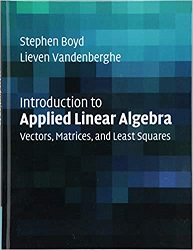Book Description

This groundbreaking textbook combines straightforward explanations with a wealth of practical examples to offer an innovative approach to teaching linear algebra. Requiring no prior knowledge of the subject, it covers the aspects of linear algebra - vectors, matrices, and least squares - that are needed for engineering applications, discussing examples across data science, machine learning and artificial intelligence, signal and image processing, tomography, navigation, control, and finance.

The numerous practical exercises throughout allow students to test their understanding and translate their knowledge into solving real-world problems, with lecture slides, additional computational exercises in Julia and MATLAB, and data sets accompanying the book online. It is suitable for both one-semester and one-quarter courses, as well as self-study, this self-contained text provides beginning students with the foundation they need to progress to more advanced study.

• Stephen Boyd is the Samsung Professor of Engineering, and Professor of Electrical Engineering at Stanford University,California, with courtesy appointments in the Department of Computer Science, and the Department of Management Science and Engineering. He is the co-author of Convex Optimization (Cambridge, 2004), written with Lieven Vandenberghe.
Reviews, Ratings, and Recommendations: Related Book Categories: Read and Download Links:Similar Books:
•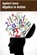Applied Linear Algebra in Action (Vasilios N. Katsikis)

This textbook contains a collection of six high-quality chapters. The techniques are illustrated by a wide sample of applications. This book is devoted to Linear Mathematics by presenting problems in Applied Linear Algebra of general or special interest.

•Applied and Computational Linear Algebra: A First Course

This book is intended as a text for a graduate course that focuses on applications of linear algebra and on the algorithms used to solve the problems that arise in those applications. It uses matrix theory to understand the workings of the algorithms.

•Linear Algebra (Jim Hefferon)

This textbook covers linear systems and Gauss' method, vector spaces, linear maps and matrices, determinants, and eigenvectors and eigenvalues. Each chapter has three or four discussions of additional topics and applications.

•Linear Algebra: A Course for Physicists and Engineers (Arak Mathai)

This textbook on linear algebra is written to be easy to digest by non-mathematicians. It introduces the concepts of vector spaces and mappings between them without too much theorems and proofs. Various applications of the formal theory are discussed as well.

•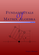Fundamentals of Matrix Algebra (Gregory Hartman)

A college (or advanced high school) level text dealing with the basic principles of matrix and linear algebra. It covers solving systems of linear equations, matrix arithmetic, the determinant, eigenvalues, and linear transformations.

•Matrix Algebra (Marco Taboga)

This is a course in matrix algebra, with a focus on concepts that are often used in probability and statistics. Matrix algebra is one of the most important areas of mathematics for data analysis and for statistical theory.

•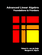Advanced Linear Algebra: Foundations to Frontiers (Robert Geijn)

The focus is on numerical linear algebra, the study of how theory, algorithms, and computer arithmetic interact. These materials keep the learner engaged by intertwining text, videos, exercises, and programming activities in consumable chunks.

•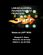Linear Algebra: Foundations to Frontiers (M. Myers, et al.)

This book is an introduction to the basic concepts of linear algebra, along with an introduction to the techniques of formal mathematics. It begins with systems of equations and matrix algebra before moving into the advanced topics.

•Computational Linear Algebra and N-dimensional Geometry

This undergraduate textbook on Linear Algebra and n-Dimensional Geometry, in a self-teaching style, is invaluable for sophomore level undergraduates in mathematics, engineering, business, and the sciences.

•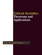Linear Algebra - Theorems and Applications (Hassan Abid Yasser)

This book contains selected topics in linear algebra, which represent the recent contributions in the most famous and widely problems. It includes a wide range of theorems and applications in different branches of linear algebra, etc.

•A First Course in Linear Algebra (Robert A Beezer)

Linear Algebra: Foundations to Frontiers (LAFF) is packed full of challenging, rewarding material that is essential for mathematicians, engineers, scientists, and anyone working with large datasets.

•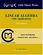Linear Algebra with Applications (W. Keith Nicholson)

The aim of the text is to achieve a balance among computational skills, theory, and applications of linear algebra. It is a relatively advanced introduction to the ideas and techniques of linear algebra targeted for science and engineering students.

Book Categories
 :All CategoriesRecent BooksMiscellaneous BooksComputer LanguagesComputer ScienceData Science/DatabasesElectrical EngineeringJava and Java EE (J2EE)Linux and UnixMathematicsMicrosoft and .NETMobile ComputingNetworking and CommunicationsSoftware EngineeringSpecial TopicsWeb Programming
Other Categories